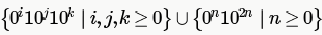# Context Free Language MCQ QUIZ - 1

## 15 Questions MCQ Test RRB JE for Computer Science Engineering | Context Free Language MCQ QUIZ - 1

Description
This mock test of Context Free Language MCQ QUIZ - 1 for Computer Science Engineering (CSE) helps you for every Computer Science Engineering (CSE) entrance exam. This contains 15 Multiple Choice Questions for Computer Science Engineering (CSE) Context Free Language MCQ QUIZ - 1 (mcq) to study with solutions a complete question bank. The solved questions answers in this Context Free Language MCQ QUIZ - 1 quiz give you a good mix of easy questions and tough questions. Computer Science Engineering (CSE) students definitely take this Context Free Language MCQ QUIZ - 1 exercise for a better result in the exam. You can find other Context Free Language MCQ QUIZ - 1 extra questions, long questions & short questions for Computer Science Engineering (CSE) on EduRev as well by searching above.
QUESTION: 1

### Which of the following statements is true?

Solution:

(A) is wrong as a language can be context free even if it is being accepted by non deterministic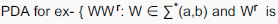reverse}

(C) and (D) not always true as Context free languages are not closed under Complement and Intersection.

QUESTION: 2

### Let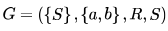be a context free grammar where the rule set R is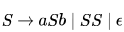Which of the following statements is true?

Solution:

It will be easy to analyze the problem if we replace terminal a and b by ( and ) respectively.

S →(S) | SS | ε

L(G) = balanced parentheses [each left parenthesis has a matching right parenthesis and are well nested ]

example () , ()(), (()), (()()()).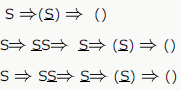String () can be derived by above three way each having different derivation tree.

So G is Ambiguous
b) Concatenation of two balance parenthesis will be balanced also . eq. x = (()) y= () xy= (())().
c) We can design Deterministic PDA for L. put left parenthesis (only) in stack and pop with right parenthesis.
d) We cannot design DFA for L because we need a stack to match left parenthesis with right parenthesis.
only option C is true.

QUESTION: 3

### Consider the languages: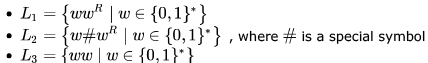Which one of the following is TRUE?

Solution:
QUESTION: 4

Let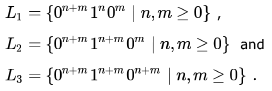Which of these languages are NOT context free?

Solution:

L1 is context-free. We count the number of 0's and check if the remaining number of 1's followed by 0's count to the initial
number of 0's.

L2 is not context-free. Here the number of 0's and the following 1's must be same, which can be checked using a PDA. But
after that we must also ensure that the following number of 0's must be less than the previous count of 0's and 1's
(otherwise n < 0, which violates the condition for acceptance) and we cannot do these two checks using a single PDA.

L3 is again not context-free as it is nothing but equal number of 0's followed by equal number of 1's followed by equal
number of 0's.

QUESTION: 5

In the context-free grammar below, S is the start symbol, a and b are terminals, and ϵ denotes the empty string.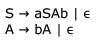The grammar generates the language

Solution: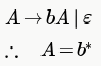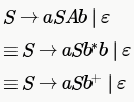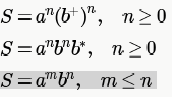QUESTION: 6

In the context-free grammar below, S is the start symbol, a and b are terminals, and ϵ denotes the empty string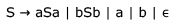Which of the following strings is NOT generated by the grammar?

Solution:

L(G) = PALINDROME
baba does not belong to palindrome , so B is the answer

QUESTION: 7

The two grammars given below generate a language over the alphabet {x, y, z}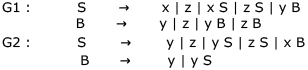Which one of the following choices describes the properties satisfied by the strings in these languages?

Solution:

from Above grammar
Regular expression for G1: (x+z)+ + (x+z)*y(y+z)+

Regular expression for G2 :(y+z+xy)+

QUESTION: 8

Consider the grammar given below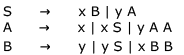Consider the following strings.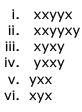Which of the above strings are generated by the grammar ?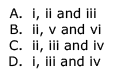Solution:

Above grammar is for equal no of x and y

from Non-terminal S -> xB

=>xy [as B->y one y for one x]

S->xB
=> xxBB [as B ->yBB one B result in one y for one x ]

S->xB
=>xyS [as B->yS one y for one x and start again]

QUESTION: 9

Consider the following grammars. Names representing terminals have been specified in capital letters.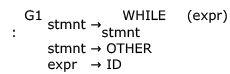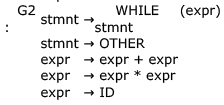Which one of the following statements is true?

Solution:

Regular grammar is either right linear or left linear. A left linear grammar is one in which there is at most 1 non-terminal on the right side of any production, and it appears at the left most position. Similarly, in right linear grammar non-terminal appears at the right most position.

Here, we can write a right linear grammar for G1 as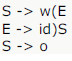(w - WHILE, o - OTHER)

So, L(G1) is regular.

Now for G2 also we can write a right linear grammar: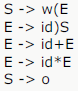making its language regular.

So, both G1 and G2 have an equivalent regular grammar. But as such both these grammars are neither right linear nor left
linear and hence not a regular grammar. So, D must be the answer.

QUESTION: 10

A CFG G is given with the following productions where S is the start symbol, A is a non-terminal and a and b are terminals.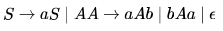Which of the following strings is generated by the grammar above?

Solution:

S→ aS
S→ aA
S→ aaAb
S→ aabAab
S→ aabbAaab
S→ aabbaab

QUESTION: 11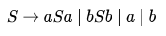The language generated by the above grammar over the alphabet  is the set of

Solution:

string generated by this language is a,b,aba,bab,aabaa,.....all this strings are odd length palindromes

QUESTION: 12

Which of the following languages are context-free?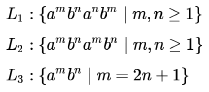Solution:

first check for L1. now look a^m&b^m and a^n&b^n must b comparable using one stack for CFL. now take a stack push all a^m in to the stack then push all b^n in to stack now a^n is coming so pop b^n for each a^n by this b^n and a^n will b comparable. now we have left only a^m in stack and b^m is coming so pop a^m for each b^m by which we can compare a^m to b^m ..we conclude that we are comparing this L1 using a single stack so this is CFG.

now for L2.this can not be done in to a single stack because m and n are not comparable we can not find when to push or pop so this is CSL.

now for L3.push all a's into stack and pop 2a 's for every b and at last we left with a single a .
bcz here aaaaabb is a valid string where m=2n+1 and n=2.So realized using single stack hence L3 is CFG.

QUESTION: 13

Consider the following context-free grammar over the alphabet ∑  = {a,b,c } with S as the start symbol: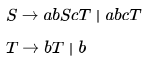Which one of the following represents the language generated by the above grammar?

Solution:

Consider the 1st production S-> abScT
This production generates equal number of (ab)'s and c's but after each c there is T which goes to T->bT

so with each c there can be one or more b's (one because of production T->b and more because of T->bT)

and these b's are independent.

For example

ababcbbcbb is the part of the langugage
and ababcbbbbbbcbb is also the part of the language so we rule out A and C both say equal number of b's after each c

In option D equal number of (ab)'s and c's is not satisfied . The only option that satisfies these 2 conditions is B

number of (ab)'s and c's is not satisfied . The only option that satisfies these 2 conditions is B

people who says this will be the string at any case

S-> abcT

-> abcb

no problem for i B in this case also here cmn=cm1 means n value 1 thats it

and remember in B c is not coming eternally.it is coming until m value reaches n of b..right there

.if m=n=1 then cb stops

QUESTION: 14

If G is a grammar with productions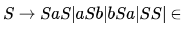where S is the start variable, then which one of the following strings is not generated by G ?

Solution:
QUESTION: 15

Consider a CFG with the following productions.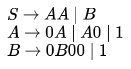S is the start symbol, A and B are non-terminals and 0 and 1 are the terminals. The language generated by this grammar is

Solution: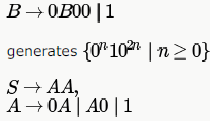generates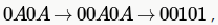which is there in only B and D choices. D is not the answer as "00" is not generated by the given grammar. So, only option left is B and if we see carefully, non-terminal B is generating the second part of B choice and AA is generating the first part.